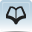# Resource 4: Times table (Example 9 times table)Teacher resource for planning or adapting to use with pupils

• 1 x 9 = 9
• 2 x 9 = 18
• 3 x 9 = 27
• 4 x 9 = 36
• 5 x 9 = 45
• 6 x 9 = 54
• 7 x 9 = 63
• 8 x 9 = 72
• 9 x 9 = 81
• 10 x 9 = 90
• 11 x 9 = 99
• 12 x 9 = 108
• 13 x 9 = 117
• 14 x 9 = 126
• #
• #
• 17 x 9 = ?

The units decrease by one each time you go down and the tens increase by one except between 10 x and 11 x

When the digits are added the sum is 9.

Resource 3: Tens and units

Resource 5: Multiplication games